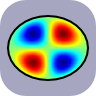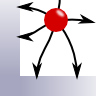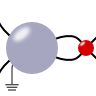Boundary Value Problems

The fundamental solution to the Poisson equation with respect to a point-like excitation is known. Such a solution is called Green's function - in three dimensions, we have the famous $$1/r$$-dependency. However, if one knows the Green's function for a differential operator (here Laplace $$\Delta$$) any problem can be solved with respect to the given boundary conditions. In this section we will learn different techniques to solve such boundary value problems, for example the method of mirror charges or direct integrations.

A Dipole in front of a flat Metallic surfaceThe Green's function can be used to find the electric field or electrostatic potential for arbitrary charge distributions. You will learn that the polarization of a dipole in front of a flat metallic surface enormously affects the overall field.

The Boundary Integral EquationThe so-called boundary integral equation relates the values of the electrostatic potential in some domain to its values at that domain's boundary. In this problem we will derive this important statement which leads to the "Boundary Element Method", a discretized version with numerical applications throughout science and engineering.

A Point Charge Close to a Grounded Metallic CornerThe method of image charges is a powerful technique to find the electrostatic potential in an intuitive way. In this problem you will find out how it can be applied to a point charge close to two grounded intersecting metallic half-planes.

A Metallic Sphere subject to a Point ChargeIn this problem we will encounter the main physical features of dielectric spheres - their induced field and polarizability. The result is of great interest: understanding the interaction of light with small particles is one of the main concerns of nanophotonics. It leads to astonishing applications like cloaking, improvement of solar cells and lithography with extreme resolution.